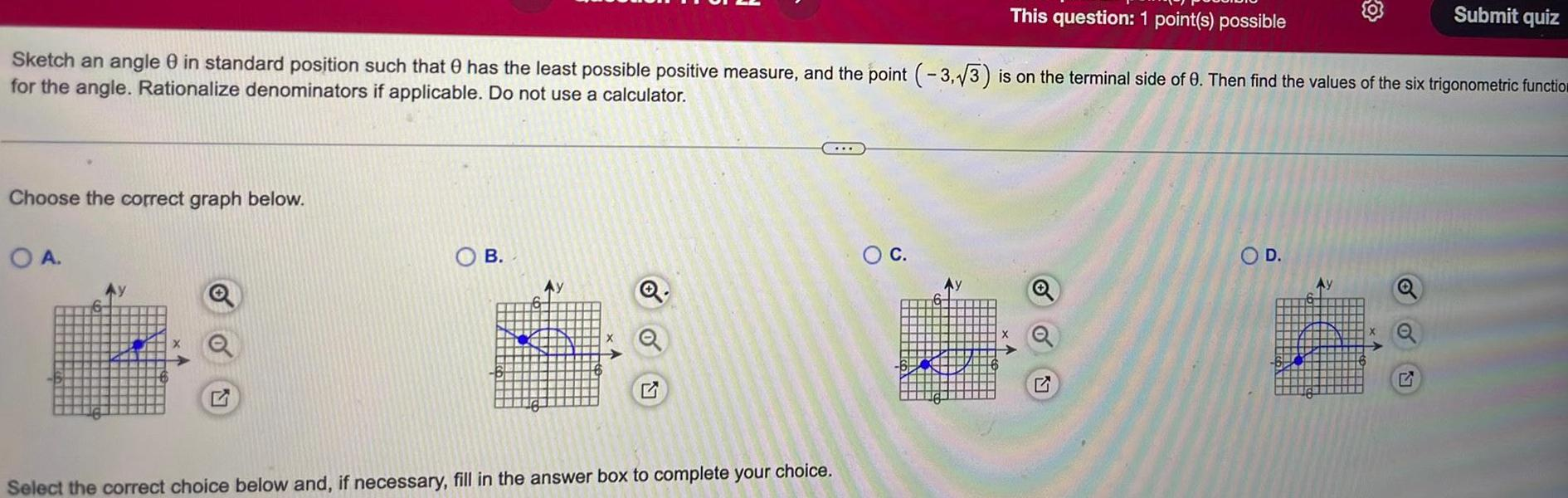Question:

# This question 1 point s possible Sketch an angle 8 in

Last updated: 9/17/2023This question 1 point s possible Sketch an angle 8 in standard position such that has the least possible positive measure and the point 3 3 is on the terminal side of 0 Then find the values of the six trigonometric functio for the angle Rationalize denominators if applicable Do not use a calculator Choose the correct graph below OA B Select the correct choice below and if necessary fill in the answer box to complete your choice O C O D Submit quiz